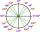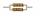Arc

Convert to arc measures (radians): 924 °

Result write as a multiple of π

Result

924° =  5.1 π

Solution:Leave us a comment of example and its solution (i.e. if it is still somewhat unclear...):Be the first to comment!Next similar examples:Convert magnitude of the angle α = 9°39'15" to radians:What is greater angle? -0.7 radians or -0.7π radians?
3. Electronics: Resistors in parallelFrom relation for calculating the resistance of parallel combination of resistors: ? Calculate the R, if R1 = 2Ω a R2 = 15Ω
4. ResistanceA resistor having an electrical resistance of 1.5 k ohms passes an electrical current of 0.1 A. Calculate what voltage is between the terminals of the resistor.
5. TreesA certain species of tree grows an average of 0.5 cm per week. Write an equation for the sequence that represents the weekly height of this tree in centimeters if the measurements begin when the tree is 200 centimeters tall.
6. Reference angleFind the reference angle of each angle:
7. SlopeFind the slope of the line: x=t and y=1+t.
8. Theorem proveWe want to prove the sentence: If the natural number n is divisible by six, then n is divisible by three. From what assumption we started?
9. Volleyball8 girls wants to play volleyball against boys. On the field at one time can be six players per team. How many initial teams of this girls may trainer to choose?
10. Nineteenth memberFind the nineteenth member of the arithmetic sequence: a1=33 d=5 find a19
11. One halfOne half of ? is: ?
12. Linear systemSolve a set of two equations of two unknowns: 1.5x+1.2y=0.6 0.8x-0.2y=2
13. Factory and divisionsThe factory consists of three auxiliary divisions total 2,406 employees. The second division has 76 employees less than 1st division and 3rd division has 212 employees more than the 2nd. How many employees has each division?
14. Two equationsSolve equations (use adding and subtracting of linear equations): -4x+11y=5 6x-11y=-5
15. PowersExpress the expression ? as the n-th power of the base 10.
16. 6 termsFind the first six terms of the sequence. a1 = 7, an = an-1 + 6
17. Functions f,gFind g(1) if g(x) = 3x - x2 Find f(5) if f(x) = x + 1/2